0.0

# Wolfram Pre-Algebra Course Assistant

• 内容摘要
Taking pre-algebra? Then you need the Wolfram Pre-Algebra Course Assistant. This definitive app for pre-algebra—from the world leader in math software—will help you work through your homework problems, ace your tests, and learn pre-algebra concepts. Forget canned examples! The Wolfram Pre-Algebra Course Assistant solves your specific pre-algebra problems on the fly.

This app covers the following pre-algebra topics:

- Find the divisors and prime factorization of a number
- Calculate the GCD and LCM of two numbers
- Determine the percent change
- Reduce and round numbers
- Evaluate expressions
- Solve equations and simplify expressions
- Convert units of length, area, volume, and weight
- Compute the mean, median, and mode of a dataset
- Plot equations on the coordinate plane
- Graph inequalities on a number line
- Calculate the area, surface area, or volume of a geometric figure
- Find the midpoint, slope, and distance between two points

The Wolfram Pre-Algebra Course Assistant is powered by the Wolfram|Alpha computational knowledge engine and is created by Wolfram Research, makers of Mathematica—the world's leading software system for mathematical research and education.

The Wolfram Pre-Algebra Course Assistant draws on the computational power of Wolfram|Alpha's supercomputers over a 3G, 4G, or Wi-Fi connection.

展开↓

• 版本中的新功能

展开↓

• 应用截图•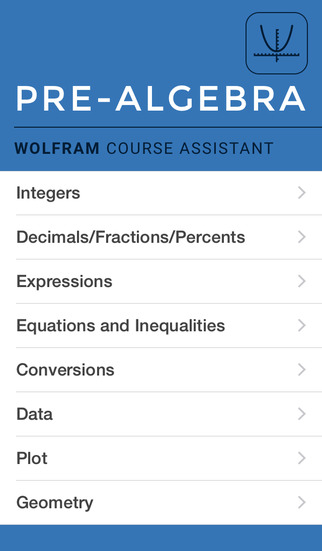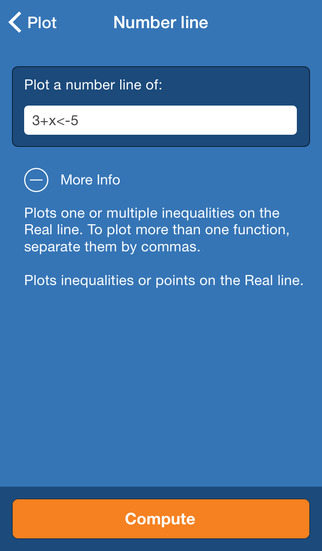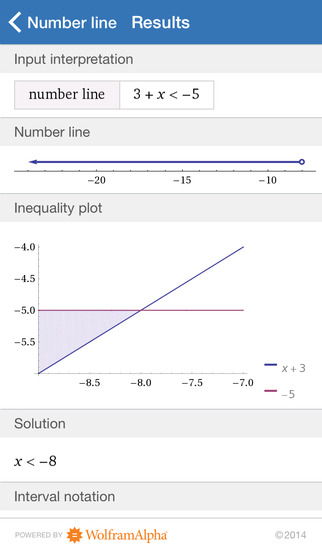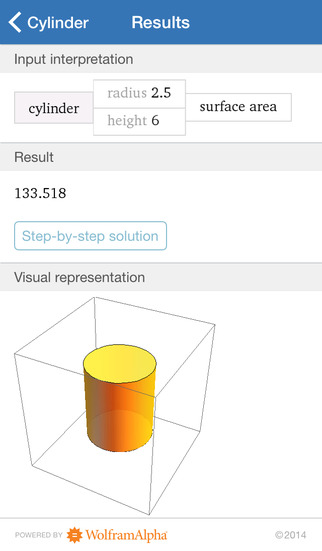•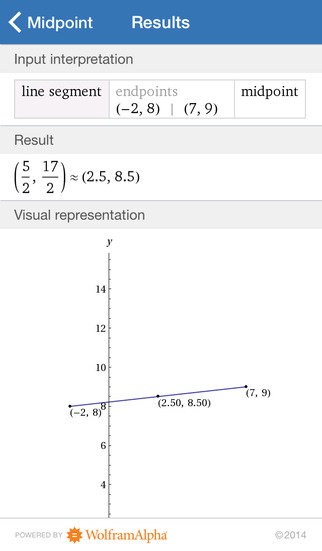#### 同类热门推荐

••AnkiMobile Flashcards 2.0.64 | 30.10MB
•••Toca Life: Hospital 1.0.1 | 220.00MB

#### 开发商的其他应用

•WolframAlpha 1.7.3 | 30.40MB